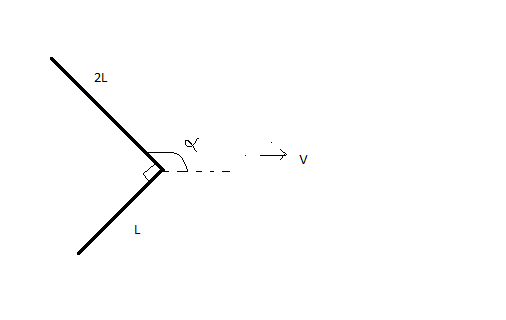Equilibrium of JEE!A straight metal rod of length $3L$ is bent through the right angle as shown. The bent rod is then placed on a rough horizontal table. A light string is attached to the vertex of the right angle. The string is then pulled horizontally so that rod slides at a constant velocity $v$ .

Find the angle $\alpha$ (in degrees) that the side $2L$ would make with the string.

Details and Assumptions

• Mass density of rod: $\rho_r= 10\text{ kg/m}$,
• Length: $L = 10\text{ m}$,
• Velocity: $v= 10 \text{ m/s}$.
×